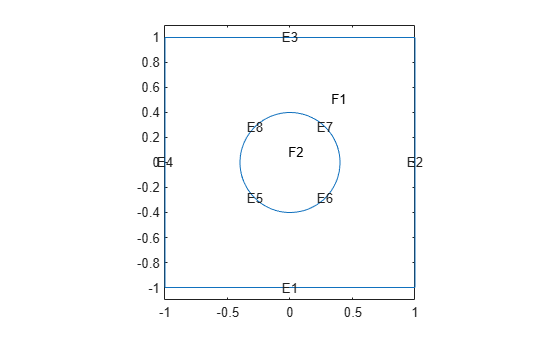## Inhomogeneous Heat Equation on Square Domain

This example shows how to solve the heat equation with a source term.

The basic heat equation with a unit source term is

`$\frac{\partial u}{\partial t}-\Delta u=1$`

This equation is solved on a square domain with a discontinuous initial condition and zero temperatures on the boundaries.

Create a transient thermal model.

`thermalmodel = createpde('thermal','transient');`

Create a square geometry centered at `x = 0` and `y = 0` with sides of length 2. Include a circle of radius 0.4 concentric with the square.

```R1 = [3;4;-1;1;1;-1;-1;-1;1;1]; C1 = [1;0;0;0.4]; C1 = [C1;zeros(length(R1) - length(C1),1)]; gd = [R1,C1]; sf = 'R1+C1'; ns = char('R1','C1')'; g = decsg(gd,sf,ns);```

Append the geometry to the model.

`geometryFromEdges(thermalmodel,g);`

Specify thermal properties of the material.

```thermalProperties(thermalmodel,'ThermalConductivity',1,... 'MassDensity',1,... 'SpecificHeat',1);```

Specify internal heat source.

`internalHeatSource(thermalmodel,1);`

Plot the geometry and display the edge labels for use in the boundary condition definition.

```figure pdegplot(thermalmodel,'EdgeLabels','on','FaceLabels','on') axis([-1.1 1.1 -1.1 1.1]); axis equal title 'Geometry With Edge and Subdomain Labels'```Set zero temperatures on all four outer edges of the square.

`thermalBC(thermalmodel,'Edge',1:4,'Temperature',0);`

The discontinuous initial value is 1 inside the circle and zero outside. Specify zero initial temperature everywhere.

`thermalIC(thermalmodel,0);`

Specify non-zero initial temperature inside the circle (Face 2).

`thermalIC(thermalmodel,1,'Face',2);`

Generate and plot a mesh.

```msh = generateMesh(thermalmodel); figure; pdemesh(thermalmodel); axis equal```Find the solution at 20 points in time between 0 and 0.1.

```nframes = 20; tlist = linspace(0,0.1,nframes); thermalmodel.SolverOptions.ReportStatistics ='on'; result = solve(thermalmodel,tlist);```
```99 successful steps 0 failed attempts 200 function evaluations 1 partial derivatives 20 LU decompositions 199 solutions of linear systems ```
`T = result.Temperature;`

Plot the solution.

```figure Tmax = max(max(T)); Tmin = min(min(T)); for j = 1:nframes pdeplot(thermalmodel,'XYData',T(:,j),'ZData',T(:,j)); caxis([Tmin Tmax]); axis([-1 1 -1 1 0 1]); Mv(j) = getframe; end```To play the animation, use the `movie(Mv,1)` command.

##### SupportGet trial now